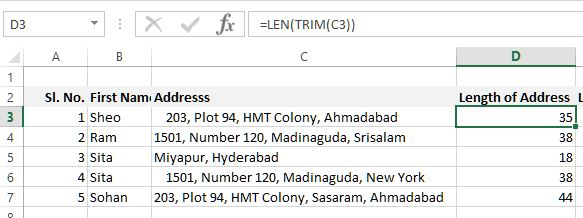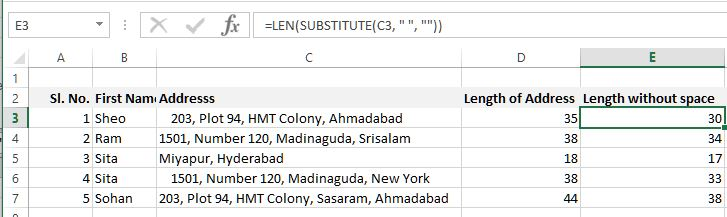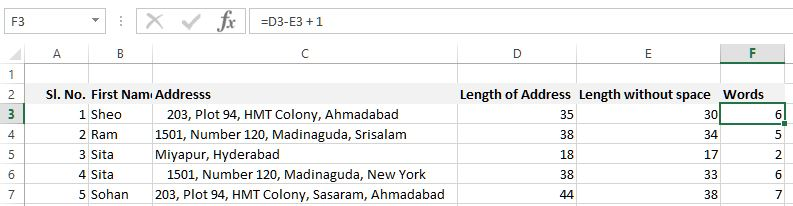# Count words in Excel

##### How to count number of words in a cell of Excel worksheet?

In the previous post, we learnt about LEFT, MID, RIGHT, FIND and SUBSTITUTE function of Excel. In this post we shall learn how to count the number of words in a cell of Excel worksheet.

Let's solve this problem three simple step.

### Step 1: Count the total length of string

To count the total length of string we shall use the LEN function of Excel. In D3 cell we have written '=LEN(TRIM(C3))' function that first trims any space before and after the cell data and then gives the length of the string.### Step 2: Count the length of string without space

To count the length of the string without space, we have entered '=LEN(SUBSTITUTE(C3, " ", ""))' formula in E3 cell that first replaces the " " (blank space) with "" (nothing) and then counts the length of string.### Step 3: Deduct the length without space from total length of string

Now deduct the length of string without space from the total length of string and add 1. Here is the correct word count in F3 cell (formula '=D3-E3 + 1').

Copy-paste the same formula to other cells and below is the result.Views: 3858 | Post Order: 24